ISEE Upper Level Math : Mode

Example Questions

← Previous 1 3

Example Question #1 : How To Find Mode

The data set below represents a class's scores on a recent math test. What is the mode score in the data set?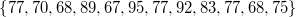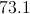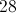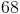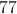Explanation:

The mode is simply the value that appears most often in a given data set. In this data set the value 77 appears three times. The value 68 appears twice. No other value appears more than once, which means 77 is the mode.

You may want to arrange the values in numerical order if it helps you see the repeating values, but it isn't required.

Example Question #1 : How To Find Mode

Examine the data set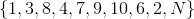. You are given thatcan be any integer from 1 to 10.

Which value(s) ofwould give the data set no mode?

Any integer from 1 to 10 except 5.

This is impossible for any value of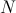.

Only 5.

Any integer from 1 to 10.

1, 3, 5, 7, or 9.

Only 5.

Explanation:

The mode of a data set is the element that appears most frequently; a data set with no mode has all of its elements appear exactly once.

All of the integers from 1 to 10 except for 5 are known to appear in this data set at least once. Ifis equal to any of those integers, it is the only one repeated, and it is the mode. Only if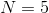can the set not have a repeated value. That makes this the correct choice.

Example Question #1 : How To Find Mode

Give the mode(s) of the data set: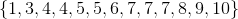The data set has no mode.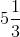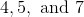Explanation:

The mode of a data set is the element that appears most frequently. In this set, that element is, which appears three times. No other element appears more than twice.

Example Question #4 : How To Find Mode

Give the mode(s) of the data set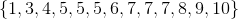The data set has no mode.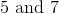Explanation:

The mode of a data set is the element that appears the most frequently. When two numbers both appear most frequently, there are two modes:Example Question #2 : How To Find Mode

Find the mode and the median of the frequency distribution shown in the following table: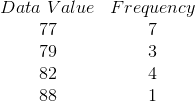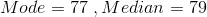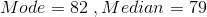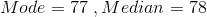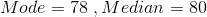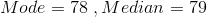Explanation:

The mode is the value that appears most often or has more frequency which is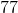in this problem. The total number of data values are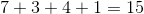. Since the number of values is odd, the median is the single middle value which is the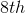value in this problem. So the median is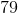.

Example Question #6 : How To Find Mode

in the following set of data the mode is, what are the possible values for.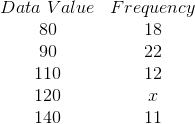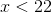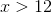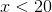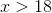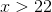Explanation:

The mode is the value that has more frequency in a given set of data than other values. Among the given frequencies in this problem,has the frequency ofwhich is more than others. Ifis the mode, its frequency must be more than.

Example Question #7 : How To Find Mode

The heights of the starting volleyball team for a high school are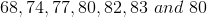inches. Give the mode of this data set.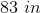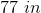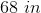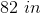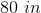Explanation:

The mode is the value that appears most often which isin this data set, becauseappears two times while all of the other values only appear one time.

Example Question #8 : How To Find Mode

In the following set of scores find the mode: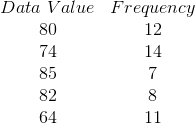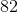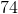Explanation:

The mode is the value that appears most often or has more frequency in a given set of data. So in this problem, the mode iswhich occurs most frequently.

Example Question #9 : How To Find Mode

Consider the data set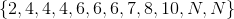.  If the data set has only one mode, how many possible values ofare there?Infinitely manyExplanation:

If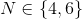then the value ofis appears five times, more than any other element. This would give the set one mode.

If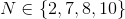, then 4, 6, and the value ofwould appear three times each, more than any other element. This would give the set three modes.

Ifassumes any other value, then 4 and 6 would appear three times each, more than any other element. This would give the set two modes.

Therefore, there are only two values ofthat would give the set one mode.

Example Question #10 : How To Find Mode

Consider the data set.  For the data set to have exactly two modes, what are all of the possible choices of?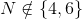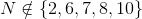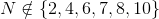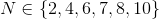Explanation:

If, then the value ofwill occur in the set five times, more than any other element. This will give the set one mode.

If, then there will be three elements -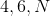- that occur three times, more than any other element. This will give the set three modes.

Ifis any other number, then there will be two elements -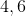- that occur twice, more than any other number. This will give the set two modes.

Therefore,, and any other value ofgives the set one mode.

← Previous 1 3